# SaturatingInductor

Simple model of an inductor with saturation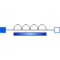# Information

This information is part of the Modelica Standard Library maintained by the Modelica Association.

This model approximates the behaviour of an inductor with the influence of saturation, i.e., the value of the inductance depends on the current flowing through the inductor. The inductance decreases as current increases.

The parameters are:

• Inom...nominal current
• Lnom...nominal inductance at nominal current
• Lzer...inductance near current = 0; Lzer has to be greater than Lnom
• Linf...inductance at large currents; Linf has to be less than Lnom

# Parameters (4)

Inom Value: Type: Current (A) Description: Nominal current Value: Type: Inductance (H) Description: Nominal inductance at Nominal current Value: Type: Inductance (H) Description: Inductance near current=0 Value: Type: Inductance (H) Description: Inductance at large currents

# Connectors (2)

p n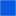Type: PositivePin Description: Positive electrical pin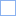Type: NegativePin Description: Negative electrical pin

# Used in Examples (1)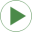ShowSaturatingInductor Modelica.Electrical.Analog.Examples Simple demo to show behaviour of SaturatingInductor component

# Used in Components (1)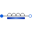SaturatingInductor Modelica.Electrical.MultiPhase.Basic Simple model of inductors with saturation